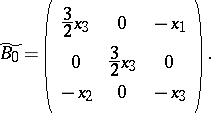# Goryachev-Chaplygin top

A rigid body rotating about a fixed point, for which:

a) the principal moments of inertia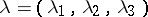, with regard to the fixed point, satisfy the relation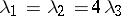;

b) the centre of mass belongs to the equatorial plane through the fixed point;

c) the principal angular momentum is perpendicular to the direction of gravity, i.e.,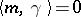. First introduced by D. Goryachev [a4] in 1900, the system was later integrated by S.A. Chaplygin [a3] in terms of hyper-elliptic integrals (cf. also Hyper-elliptic integral). The system merely satisfying a) and b) is not algebraically integrable, but on the locus, defined by c), it is; namely, it has an extra invariant of homogeneous degree: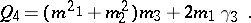C. Bechlivanidis and P. van Moerbeke [a1] have shown that the problem has asymptotic solutions which are meromorphic in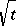; the system linearizes on a double cover of a hyper-elliptic Jacobian (i.e., of the Jacobi variety of a hyper-elliptic curve; cf. also Plane real algebraic curve), ramified exactly along the two hyper-elliptic curves, where the phase variables blow up; see also [a5]. An elementary algebraic mapping transforms the Goryachev–Chaplygin equations into equations closely related to the-body Toda lattice. A Lax pair is given in [a2]: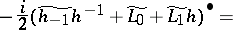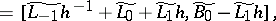where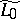and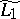are given by the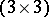right-lower corner of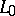and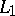and where